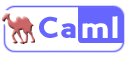# OCaml

Appeared in:
1996
Influenced by:
Influenced:
Typing discipline:
File extensions:
.ml .mli
Versions and implementations (Collapse all | Expand all):
Programming language

OCaml is a functional language developed by Inria. The language is strongly typed, but uses type inference rather than explicit variable types. For objects, a structural typing system is used. It has a very fast garbage collector built-in, a native-code and byte-code generator, a top level for entering language constructs directly into an interpreter, and very powerful pattern matching.

## Elements of syntax:

Nestable comments (* *) Yes [_a-z][_a-zA-Z0-9']* [_a-z][_a-zA-Z0-9']* let variable = value {toplevel} or let variable = value in ... {local scope} begin ... end or ( ... ) == != = <> < > <= >= let f p1 p2 ... = ... or let f = fun p1 p2 ... -> ... f a b ... f () ; if condition then ... if condition then ... else ... while true do ... done while condition do ... done for i = 1 to 10 do ... done for i = 10 downto 1 do ... doneOCaml logo

## Examples:

### Hello, World!:

Example for versions OCaml 3.11

`print_endline` is a built-in function defined with the following type:

``````string -> unit = <func>
``````

This means that it takes 1 string as a parameter, and returns the unit type, `()`.

``````let () = print_endline "Hello World";;
``````

### Factorial:

Example for versions OCaml 3.11

This example uses an auxiliary function `fact`, so that tail recursion is possible.

``````let rec fact n accum =
if n <= 1 then
accum
else
fact (n-1) (accum*n);;

let factorial n =
fact n 1;;

let () =
for n = 0 to 16 do
Printf.printf "%d! = %d\n" n (factorial n)
done;
``````

### Factorial:

Example for versions OCaml 3.11

This example shows the naive way to implement the factorial function. However, it is not tail recursive, since the recursive function call is not the only statement on the line.

``````let rec factorial n =
if n <= 1 then
1
else
factorial (n-1) * n;;

let () =
for n = 0 to 16 do
Printf.printf "%d! = %d\n" n (factorial n)
done;
``````

### Fibonacci numbers:

Example for versions OCaml 3.11

This example uses straightforward recursive solution. `Printf.printf` does formatted output.

``````let rec fibonacci n =
if n < 3 then
1
else
fibonacci (n-1) + fibonacci (n-2)

let () =
for n = 1 to 16 do
Printf.printf "%d, " (fibonacci n)
done;
print_endline "..."
``````

Example for versions OCaml 3.11

``````let square x = x *. x;;

let delta  a b c = ( square b-. (4. *. a *. c ));;

let   solve a b c   =
if a=0.
then Printf.printf "Not a quadratic equation\n"
else
if   delta a b c  >= 0.
then
let   x1=( -.b +. sqrt(delta a b c )) /. (2. *. a)
and x2=  (-.b -.  sqrt(delta a b c ))  /. (2. *. a)
in
Printf.printf "x1 =%.5f x2=%.5f \n"  x1  x2
else
let   x= (-.b   /. (2. *. a))
and i=     sqrt(4. *. a *. c -. square b)  /. (2. *. a)
in Printf.printf "x+ =%.5f+i%.5f  x-=%.5f-i%.5f \n"  x  i  x i
;;

let () =
solve (float_of_string Sys.argv.(1))( float_of_string Sys.argv.(2) )(float_of_string Sys.argv.(3))
``````